## Beverton-Holt fitting example

Here are some examples of fitting a Beverton-Holt model (a saturating hyperbolic model of the basic form $$y = x/(1+x)$$, also known as Michaelis-Menten; Monod; Holling type II; … ?) to data. It can be done via any nonlinear/maximum likelihood fitting routine, but with a bit of a trick you can also do it via glm(), which allows it be put automatically into a ggplot-based figure …

### Example 1

The data and question come from Holly Kindsvater: they're from Hocking MD and Reimchen TE. 2009. Salmon species, density, and watershed size predict magnitude of marine enrichment in riparian food webs. Oikos 118:1307-1318 (Table 1). $$X$$ is salmon spawning biomass (kg/m); $$Y$$ is the $$\delta^{15}N$$ index (isotopic signature). Other than that, I don't know anything about the biological context.

load("bevholt_ex.RData")
library(bbmle)

## Loading required package: stats4


Here's (one parameterization of) the Beverton-Holt function, in which $$a$$ is the asymptote ($$\lim_{x \to \infty} f(x)$$) and $$b$$ is the half-maximum. (Since this model has two parameters and three places you could put them ($$\bullet x/(\bullet + \bullet x)$$), and each parameter could be specified as $$c$$ or $$1/c$$, there are at least 8 ways to parameterize the model … alternatively, one could specify the asymptote, half-maximum, initial slope, or reciprocals of any of them …)

bevholt = function(S, a, b) {
S * a/(b + S)
}


Preliminary view of the data along with initial guess:

par(las = 1, bty = "l")
plot(Y ~ X, data = dat)
maxY <- max(dat$Y) curve(bevholt(x, a = maxY, b = maxY), add = TRUE)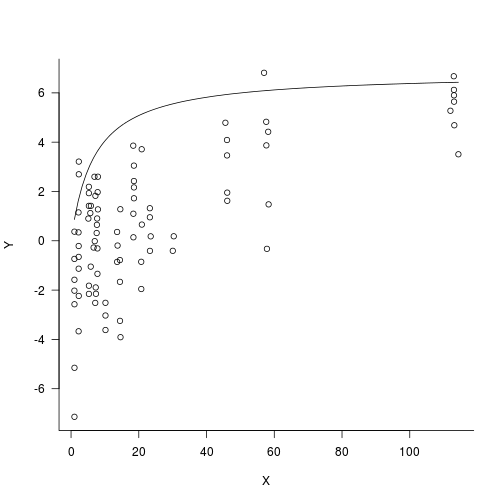We might already guess that this fit will be fairly poorly determined because both the asymptote and the half-maximum will be large (i.e. the fit may be pretty close to linear over the range of the data …) If we were just approaching this as a MLE/non-linear least squares problem we might run into trouble: nls(Y ~ bevholt(X, a, b), start = list(a = maxY, b = maxY), data = dat)  ## Error: singular gradient  If we use bbmle::mle2 it might be a little more robust, but we still run into convergence failures fit2 <- mle2(Y ~ dnorm(mean = bevholt(X, a, b), sd = exp(logsd)), data = dat, start = list(a = maxY, b = maxY, logsd = 0))  ## Warning: convergence failure: code=1 (iteration limit 'maxit' reached)  Increasing the number of iterations (e.g. control=list(maxit=10000)) or using other optimizers doesn't really help much; we'll see why shortly. coef(fit2)  ## a b logsd ## 12.1243 164.4537 0.7619  We see that as previously hinted both the asymptote $$a$$ and the half-maximum $$b$$ are estimated as being well beyond the range of the data: the best fit is very close to a straight line, which would correspond to making both the asymptote and the half-maximum infinite … par(las = 1, bty = "l") plot(Y ~ X, data = dat) with(as.list(coef(fit2)), curve(bevholt(x, a = a, b = b), lwd = 2, add = TRUE))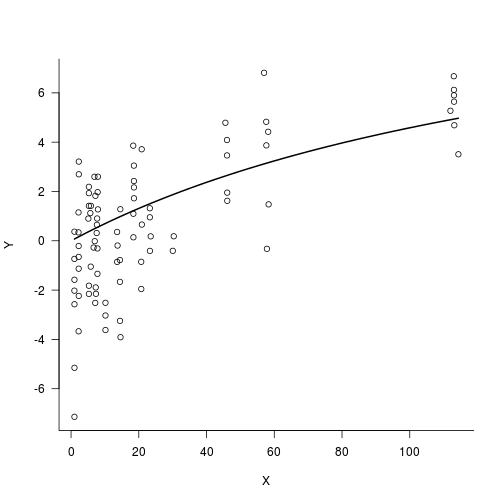We can see a very slight curvature, but this is very close to straight – and we can't really tell whether a straight line would be better, since the parameters would have to be infinite and the optimizer can't really handle that. One alternative would be to fit a straight line and compare likelihoods: fit2L <- mle2(Y ~ dnorm(mean = c + d * X, sd = exp(logsd)), data = dat, start = list(c = 0, d = 0.01, logsd = 0)) fit2L0 <- mle2(Y ~ dnorm(mean = d * X, sd = exp(logsd)), data = dat, start = list(d = 0.01, logsd = 0)) -logLik(fit2)  ## 'log Lik.' 187.7 (df=3)  -logLik(fit2L)  ## 'log Lik.' 183.1 (df=3)  -logLik(fit2L0)  ## 'log Lik.' 186.2 (df=2)  It looks like the linear model is actually better (the negative log-likelihood is about 3 units lower). However, the main reason for the improvement is that the linear model allows the intercept to be non-zero: if we force the intercept to be zero, then the linear fit is only slightly better than the Beverton-Holt (but with one less parameter). We could also have used lm(), of course, but sometimes when comparing different fitting methods it's hard to figure out whether the log-likelihoods are really using the same additive constants or not. We can also reparameterize the model so that at least one of the parameters is 0, rather than infinite, in the limit of a straight line. For example, if $$y=x/(ex+f)$$, then $$e$$ is the reciprocal of the asymptote and $$f$$ is the reciprocal of the initial slope (it will turn out that this is a useful parameterization for other reasons as well): fit3 <- mle2(Y ~ dnorm(mean = X/(e * X + f), sd = exp(logsd)), data = dat, start = list(e = 0.1, f = 1, logsd = 1)) -logLik(fit3)  ## 'log Lik.' 186.1 (df=3)  We get a log-likelihood that is very slightly better than the linear model through the origin, but the reciprocal-asymptote parameter is actually negative: coef(fit3)  ## e f logsd ## -0.02049 22.49721 0.74536  This may or may not make any biological sense (it implies that the function goes through a singularity at $$x=-f/e=1098$$) We can constrain $$e$$ to be $$\geq 0$$: fit4 <- update(fit3, lower = c(a = 0, b = 0, logsd = -Inf), method = "L-BFGS-B")  Here's the comparison – it's worth keeping in mind that none of these curves is likely to be “significantly” different in the non-statistical sense: par(las = 1, bty = "l") plot(Y ~ X, data = dat) with(as.list(coef(fit2)), curve(bevholt(x, a = a, b = b), add = TRUE)) with(as.list(coef(fit2L)), curve(c + d * x, col = 2, add = TRUE)) with(as.list(coef(fit2L0)), curve(d * x, col = 4, add = TRUE)) with(as.list(coef(fit3)), curve(x/(e * x + f), col = 5, add = TRUE)) with(as.list(coef(fit4)), curve(x/(e * x + f), col = 6, add = TRUE))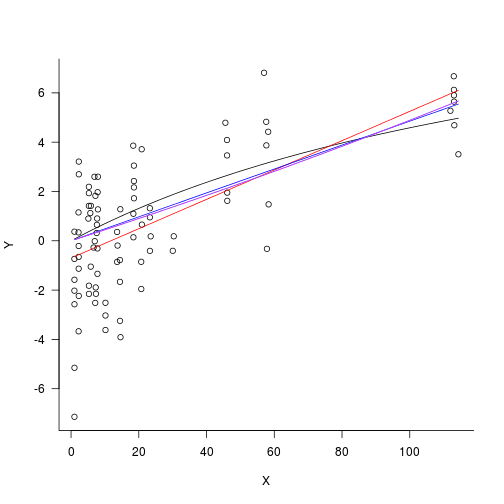(the red line is the linear fit that is allowed to have a non-zero intercept). AICtab(fit2, fit2L, fit2L0, fit3, fit4)  ## dAIC df ## fit2L 0.0 3 ## fit2L0 4.1 2 ## fit4 6.0 3 ## fit3 6.0 3 ## fit2 9.2 3  But what if we want confidence intervals? Getting confidence intervals on a nonlinear fit is a bit of a nuisance (we can use the delta method, which may under some circumstances be an unreliable approximation, or bootstrapping or MCMC, which may be nuisances to implement). In principle we can fit the last form of the Beverton-Holt shown above ($$y=x/(ex+f)$$) by using a Gaussian GLM with an inverse link and a response variable of $$1/x$$, i.e. $$1/y \sim \text{Normal}(\mu=e+f\cdot(1/x))$$: g1 <- glm(Y ~ I(1/X), family = gaussian(link = "inverse"), data = dat)  However, this doesn't actually work: coef(g1)  ## (Intercept) I(1/X) ## 1.700 -2.041  It's OK if we start from the results of fit3: coef(g2 <- glm(Y ~ I(1/X), family = gaussian(link = "inverse"), data = dat, start = coef(fit3)[1:2]))  ## (Intercept) I(1/X) ## -0.02112 22.56702  Or even from the starting values that we used for fit3: coef(g2B <- glm(Y ~ I(1/X), family = gaussian(link = "inverse"), data = dat, start = c(0.01, 1)))  ## (Intercept) I(1/X) ## -0.02112 22.56697  The convenience of doing things this way is that now ggplot2 can automatically add the curve to a plot, with error bars: library(ggplot2) theme_set(theme_bw()) p1 <- ggplot(dat, aes(X, Y)) + theme_bw() p1 + geom_point() + geom_smooth(method = "glm", family = gaussian(link = "inverse"), formula = y ~ I(1/x), start = c(0.01, 1))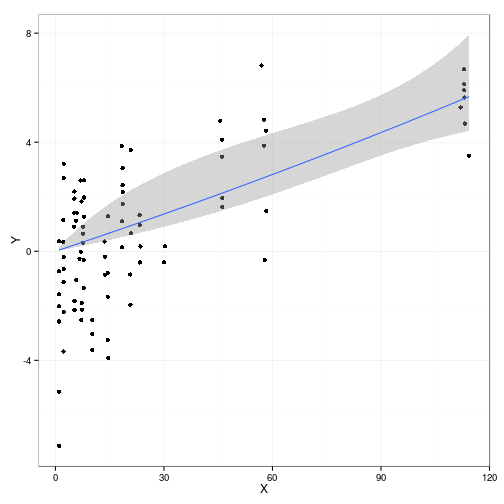## Other possibilities • explore whether the 'bad' glm() fit is a local maximum (e.g. via emdbook::slice())? • see whether glm2::glm2() does better with unspecified starting points? How about nls2, minpack.lm ? glm2 gets the same bad answer as glm, although at least we get a warning message: coef(g3 <- glm2::glm2(Y ~ I(1/X), family = gaussian(link = "inverse"), data = dat))  ## (Intercept) I(1/X) ## 1.700 -2.041  -logLik(g3)  ## 'log Lik.' 204.5 (df=3)  -logLik(g2)  ## 'log Lik.' 186.1 (df=3)  What does the likelihood/SSQ surface look like? ## dnorm with sd profiled out dnorm2 <- function(x, mean, log = FALSE) { ssq <- sum((x - mean)^2) dnorm(x, mean, sd = sqrt(ssq/length(x)), log = log) } ff <- function(e, f, X = dat$X, Y = dat$Y) { mu <- e + f * (1/X) pred <- 1/mu -sum(dnorm2(Y, mean = pred, log = TRUE)) }  This agrees with the previous fit: do.call(ff, as.list(coef(fit3)[1:2]))  ##  186.1  -logLik(fit3)  ## 'log Lik.' 186.1 (df=3)  We can draw a likelihood surface to see what's going wrong with the GLM (or MLE) fits, picking a range that includes both the 'bad' and 'good' fits: library(emdbook) par(las = 1) cc <- curve3d(ff(x, y), xlim = c(-0.5, 2), ylim = c(-5, 25), sys3d = "image", useRaster = TRUE, col = gray((100:1)/100), xlab = "e", ylab = "f") with(cc, contour(x, y, z, add = TRUE)) points(coef(g1), coef(g1), pch = 16, col = 4) points(coef(g2), coef(g2), pch = 16, col = 5) points(0, 0, col = 2, pch = 16) points(0.1, 1, col = 6, pch = 16) tred <- adjustcolor("red", alpha = 0.5) curve(-x, add = TRUE, lwd = 3, col = tred) curve(-114 * x, add = TRUE, lwd = 3, col = tred) legend("topright", c("good fit", "bad fit", "GLM start", "MLE start"), pch = 16, col = c(5, 4, 2, 6))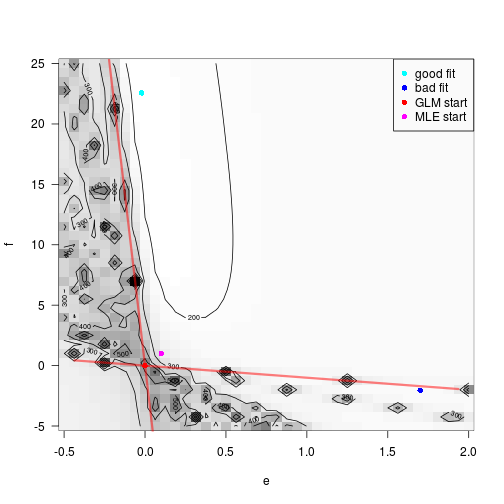We know we're in trouble whenever $$1/y = e+(f/x) \approx 0$$ for any value of $$x$$ in the data set, which corresponds to $$f=-ex$$. The range of $$x$$ is {1,114}: the red lines show the curves corresponding to the extreme values, which show exactly the range where we have trouble. If we start inside this zone it's likely that we'll end up somewhere arbitrary. By luck, the starting value for the MLE was just outside this zone … So despite the general cleverness of GLM, it's not necessarily easier/more robust for this case. It is apparently possible to fit a GLM with inequality constraints, supposedly one goes to p. 445 of Venables and Ripley. That page isn't shown in Google Books, and I don't have a copy with me at the moment … but since this is apparently an example of fitting a GLM via direct maximization rather IRLS, it may not be that much better than what I've already shown above using bbmle::mle2. ### Example 2 From Holly again: $$X$$=salmon density (kg/m2), $$Y$$=number of salmon captured by brown bears: dat2 <- setNames(read.csv("gende&quinn_1.csv", header = FALSE), c("X", "Y")) par(las = 1, bty = "l") plot(Y ~ X, data = dat2) curve(x/((1/50) + 0.01 * x), add = TRUE)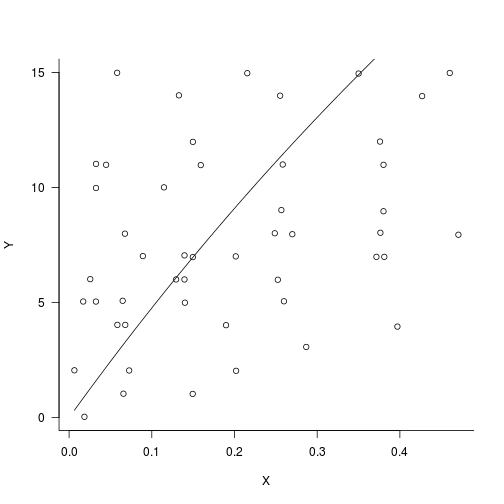Let's say this is a straight line with an nearly infinite asymptote ($$e \approx 0$$) and initial slope approximately 50 ($$f \approx 1/50$$) I tried to use method="L-BFGS-B" again to impose bounds, but it complained, so I used bobyqa from the optimx/minqa packages: library(optimx) library(minqa) d2fit1 <- mle2(Y ~ dnorm2(mean = X/(e * X + f)), data = dat2, start = list(e = 0.01, f = 1/50), optimizer = "optimx", method = "bobyqa", lower = c(e = 0, f = 0))  Check out the likelihood surface (not really necessary; I had some initial confusion over whether $$f$$ was the slope or the reciprocal slope, which had me plotting the likelihood surface to see what was going on …) cc2 <- curve3d(ff(x, y, X = dat2$X, Y = dat2$Y), xlim = c(0, 0.2), ylim = c(0, 0.1), sys3d = "image", useRaster = TRUE, col = gray((100:1)/100), xlab = "e", ylab = "f") with(cc2, contour(x, y, z, add = TRUE)) points(coef(d2fit1), coef(d2fit1), col = 4, pch = 16) points(0.01, 1/50, col = 2, pch = 16)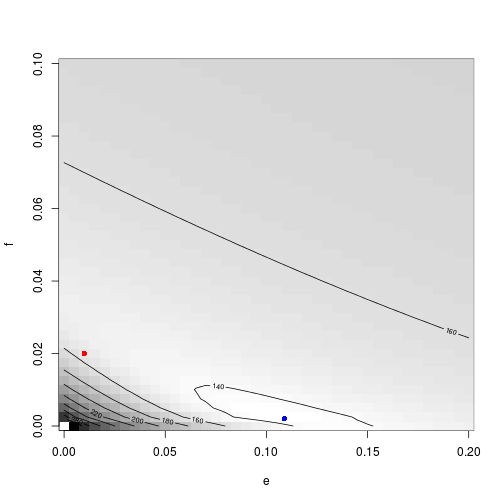Check out the fit in base graphics: par(las = 1, bty = "l") plot(Y ~ X, data = dat2) with(as.list(coef(d2fit1)), curve(x/(e * x + f), add = TRUE))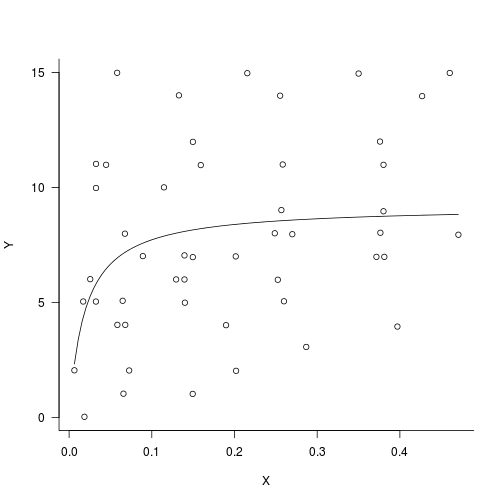Since we now know where to tell glm() where to start we can get ggplot2 to do it: ggplot(dat2, aes(X, Y)) + theme_bw() + geom_point() + geom_smooth(method = "glm", formula = y ~ I(1/x), family = gaussian(link = "inverse"), start = coef(d2fit1)[1:2])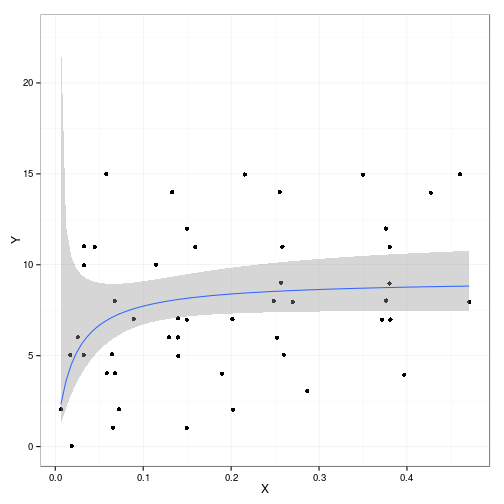If we needed to generate the confidence intervals and plot themselves, we would use the approach used by glm()/ggplot(), which is: (1) compute the variances of the predicted values via $$X^T V X$$, where $$X$$ is the model matrix of the predictor variables for the desired prediction and $$V$$ is the variance-covariance matrix of the parameters; (2) compute the confidence intervals of the predictions on the linear predictor scale via Normal approximation (i.e. $$\hat x \pm 1.96 \sigma$$); see an example of this general approach on the GLMM FAQ back-transform the confidence intervals to the raw scale; (4) use geom_ribbon() with no border line (color=NA outside of aes()) and alpha set accordingly to add the ribbon to the plot. Similarly difficult fitting was done by Poulsen et al.: Poulsen, J. R., C. W. Osenberg, C. J. Clark, D. J. Levey, and B. M. Bolker. 2007. “Plants as Reef Fish: Fitting the Functional Form of Seedling Recruitment.” The American Naturalist 170 (2) (August): 167–183. doi:10.1086/518945. JSTOR. If I were trying to implement an automatic (self-starting) algorithm that would succeed on as many noisy/small B-H data sets as possible, I would probably try some combination of • constraining the fit to non-negative parameter values; • choosing the most robust parameterization (I think $$y=X/(eX+f)$$ is not too bad and matches the inverse-link GLM, although it might be good to use $$X/(eX+1/g)$$ instead so that $$g=0$$ corresponded to an infinite initial slope (which would give a flat, non-zero line); • using the most robust optimizer that allows box constraints (BOBYQA is not too bad; there are another couple of options such as an alternate BOBYQA implementation in the nloptwrap package; an AD Model Builder solution would be pretty easy to implement for this simple case too); • finding the best way to get automatic starting values (e.g. maybe try linear regression on a subset of the data, taking the mean of a subset of the data, and a glm2 inverse-link fit, and use the best combination of those approaches). ### Example 3 It gets worse. Even if glm() reaches a plausible (positive-coefficient) answer, the confidence intervals can include implausible values. dat3 <- setNames(read.csv("nagasaka.csv", header = FALSE), c("X", "Y")) g3 <- ggplot(dat3, aes(X, Y)) + geom_point() g3 + geom_smooth(method = "glm", formula = y ~ I(1/x), family = gaussian(link = "inverse"), start = c(0.2, 0.01), fill = "blue", alpha = 0.2) + geom_smooth(method = "loess", color = "red", fill = "red", alpha = 0.2) + coord_cartesian(ylim = c(-4, 10))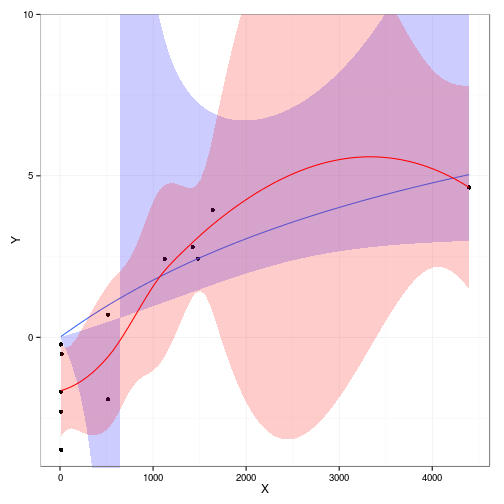(g4 <- glm(Y ~ I(1/X), family = gaussian(link = "inverse"), data = dat3, start = c(0.2, 0.01)))  ## ## Call: glm(formula = Y ~ I(1/X), family = gaussian(link = "inverse"), ## data = dat3, start = c(0.2, 0.01)) ## ## Coefficients: ## (Intercept) I(1/X) ## 0.0907 473.8105 ## ## Degrees of Freedom: 11 Total (i.e. Null); 10 Residual ## Null Deviance: 77.6 ## Residual Deviance: 31.9 AIC: 51.8  pframe <- with(dat3, data.frame(X = seq(min(X), max(X), length = 101))) pred <- predict(g4, newdata = pframe, se.fit = TRUE) minval <- 1e-06 cc <- qnorm(0.975) invlink <- g4$family$linkinv pframe <- with(pred, transform(pframe, Y = invlink(fit), lwr = invlink(fit - cc * se.fit), clwr = invlink(pmax(minval, fit - cc * se.fit)), upr = invlink(fit + cc * se.fit))) g3 + geom_line(data = pframe) + geom_ribbon(data = pframe, aes(ymin = lwr, ymax = upr), colour = NA, alpha = 0.2) + geom_ribbon(data = pframe, aes(ymin = clwr, ymax = upr), fill = "blue", colour = NA, alpha = 0.2) + coord_cartesian(ylim = c(-4, 10))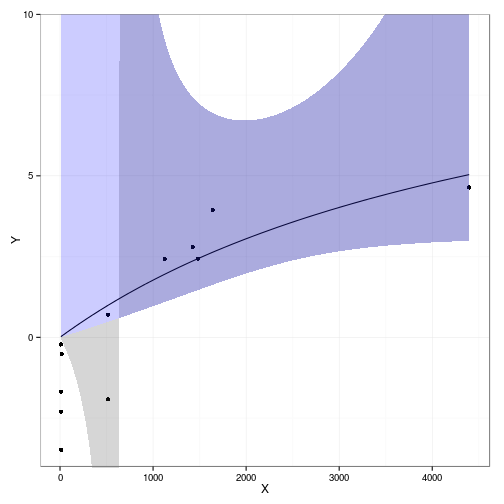cc3 <- curve3d(ff(x, y, X = dat3$X, Y = dat3$Y), xlim = c(0, 0.8), ylim = c(100, 2500), sys3d = "image", useRaster = TRUE, n = c(71, 71), col = gray((100:1)/100), xlab = "e", ylab = "f") with(cc3, contour(x, y, z, add = TRUE, levels = min(cc3$z) + qchisq(c(0.9, 0.95,
0.975), 2)/2))
points(coef(g4), coef(g4), col = 4, pch = 16, cex = 2)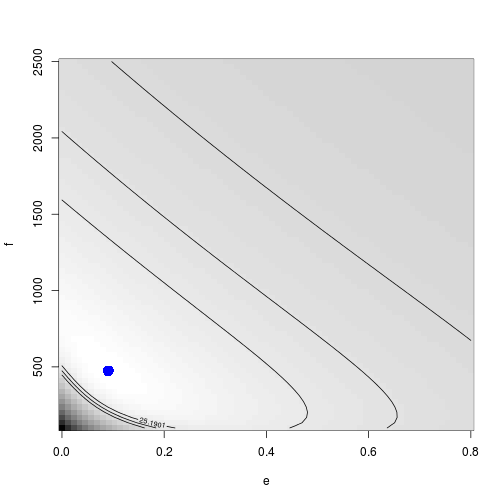The figure shows the nominal 90%, 95% and 97.5% LRT confidence intervals – as we will see, these are incorrect (too conservative) because part of the area gets cut off at the zero boundaries, so we have to include more area in the feasible region.

Sample from a quasi-Bayesian posterior density (sample automatically rescales its weights to sum to 1.0):

set.seed(101)
ss <- sample(seq_along(cc3$z), prob = exp(-cc3$z), size = 1000, replace = TRUE)

## Warning: Walker's alias method used: results are different from R < 2.2.0

ind2vals <- function(k) {
i <- k%/%ncol(cc3$z) + 1 j <- k%%ncol(cc3$z) + 1
c(cc3$x[i], cc3$y[j])
}
samp <- t(sapply(ss, ind2vals))

image(cc3, xlim = c(0, 0.8), ylim = c(100, 2500), useRaster = TRUE, col = gray((100:1)/100),
xlab = "e", ylab = "f")
with(cc3, contour(x, y, z, level = min(cc3$z) + qchisq(c(0.9, 0.95, 0.975), 2)/2, add = TRUE)) points(samp[, 1], samp[, 2], col = adjustcolor("red", alpha = 0.2), pch = 16, cex = 1.5) points(coef(g4), coef(g4), col = 4, pch = 16, cex = 2)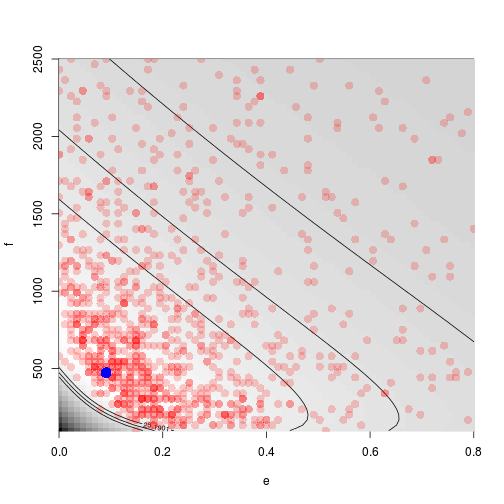pframe2 <- pframe allpred <- apply(samp, 1, function(cc) { 1/(cc + cc/pframe2$X)
})
pframe2[, c("lwr", "upr")] <- setNames(t(apply(allpred, 1, quantile, c(0.025,
0.975))), c("lwr", "upr"))
g3 + geom_line(data = pframe2) + geom_ribbon(data = pframe2, aes(ymin = lwr,
ymax = upr), colour = NA, alpha = 0.2) + coord_cartesian(ylim = c(-4, 10))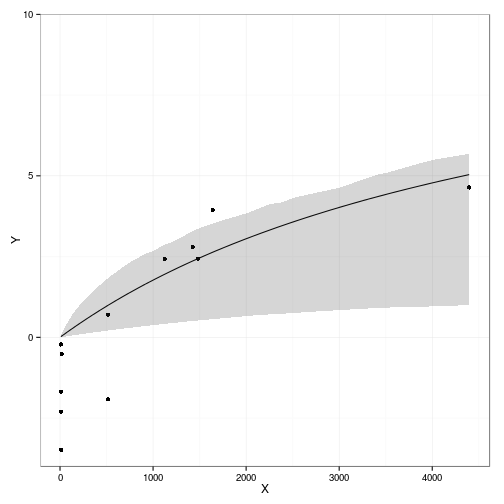Alternatively we could do this by real MCMC, either with BUGS or with MCMCpack

### Example 4

dat4 <- na.omit(setNames(read.csv("Hocking_Reynolds_d15N_blueberry.csv", header = FALSE),
c("X", "Y")))
p4 <- qplot(X, Y, data = dat4) + geom_smooth(method = "glm", family = gaussian(link = "inverse"))
coef(glm(Y ~ X, data = dat4, family = gaussian(link = "inverse")))

## (Intercept)           X
##     0.22934    -0.04653

d4fit1 <- mle2(Y ~ dnorm2(mean = X/(e * X + f)), data = dat4, start = list(e = 0.01,
f = 1/50), optimizer = "optimx", method = "bobyqa", lower = c(e = 1e-06,
f = 1e-06))
pframe <- with(dat4, data.frame(X = seq(min(X), max(X), length = 101)))
pframe$Y <- with(c(as.list(coef(d4fit1)), pframe), X/(e * X + f)) p4 + geom_line(data = pframe, colour = "red")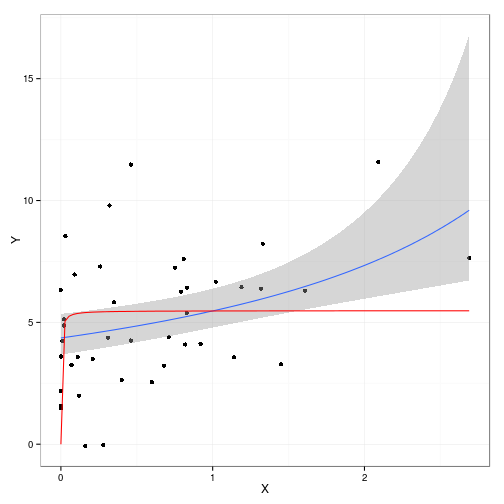Since geom_smooth(method="glm",...) doesn't work here, we'd have to do something else to get the confidence intervals cc4 <- curve3d(ff(x, y, X = dat4$X, Y = dat4$Y), xlim = c(0.1, 0.3), ylim = c(1e-06, 0.03), sys3d = "image", useRaster = TRUE, n = c(71, 71), col = gray((100:1)/100), xlab = "e", ylab = "f") with(cc4, contour(x, y, z, add = TRUE, levels = min(cc4$z) + qchisq(c(0.9, 0.95,
0.975), 2)/2, labels = c(0.9, 0.95, 0.975)))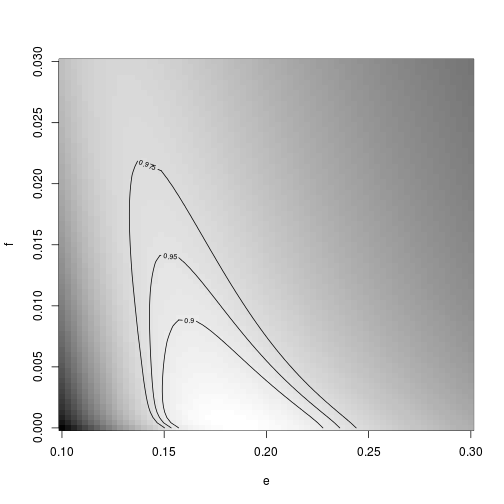et cetera …